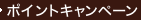•### Uncertainty Analysis with High Dimensional Dependence Modelling

• 在庫がございません。海外の書籍取次会社を通じて出版社等からお取り寄せいたします。
通常6～9週間ほどで発送の見込みですが、商品によってはさらに時間がかかることもございます。
重要ご説明事項
1. 納期遅延や、ご入手不能となる場合がございます。
2. 複数冊ご注文の場合、分割発送となる場合がございます。
3. 美品のご指定は承りかねます。
• ≪洋書のご注文につきまして≫ 「海外取次在庫あり」および「国内仕入れ先からお取り寄せいたします」表示の商品でも、納期の目安期間内にお届けできないことがございます。あらかじめご了承ください。

• 製本 Hardcover:ハードカバー版／ページ数 284 p.
• 言語 ENG
• 商品コード 9780470863060
• DDC分類 003.54

### Full Description

Mathematical models are used to simulate complex real-world phenomena in many areas of science and technology. Large complex models typically require inputs whose values are not known with certainty. Uncertainty analysis aims to quantify the overall uncertainty within a model, in order to support problem owners in model-based decision-making. In recent years there has been an explosion of interest in uncertainty analysis. Uncertainty and dependence elicitation, dependence modelling, model inference, efficient sampling, screening and sensitivity analysis, and probabilistic inversion are among the active research areas. This text provides both the mathematical foundations and practical applications in this rapidly expanding area, including:* An up-to-date, comprehensive overview of the foundations and applications of uncertainty analysis.* All the key topics, including uncertainty elicitation, dependence modelling, sensitivity analysis and probabilistic inversion.* Numerous worked examples and applications.* Workbook problems, enabling use for teaching.* Software support for the examples, using UNICORN - a Windows-based uncertainty modelling package developed by the authors.*A website featuring a version of the UNICORN software tailored specifically for the book, as well as computer programs and data sets to support the examples. Uncertainty Analysis with High Dimensional Dependence Modelling offers a comprehensive exploration of a new emerging field. It will prove an invaluable text for researches, practitioners and graduate students in areas ranging from statistics and engineering to reliability and environmetrics.

### Contents

Preface. 1 Introduction. 1.1 Wags and Bogsats. 1.2 Uncertainty analysis and decision support: a recent example. 1.3 Outline of the book. 2 Assessing Uncertainty on Model Input. 2.1 Introduction. 2.2 Structured expert judgment in outline. 2.3 Assessing distributions of continuous univariate uncertain quantities. 2.4 Assessing dependencies. 2.5 Unicorn. 2.6 Unicorn projects. 3 Bivariate Dependence. 3.1 Introduction. 3.2 Measures of dependence. 3.3 Partial, conditional and multiple correlations. 3.4 Copulae. 3.5 Bivariate normal distribution. 3.6 Multivariate extensions. 3.7 Conclusions. 3.8 Unicorn projects. 3.9 Exercises. 3.10 Supplement. 4 High-dimensional Dependence Modelling. 4.1 Introduction. 4.2 Joint normal transform. 4.3 Dependence trees. 4.4 Dependence vines. 4.5 Vines and positive definiteness. 4.6 Conclusions. 4.7 Unicorn projects. 4.8 Exercises. 4.9 Supplement. 5 Other Graphical Models. 5.1 Introduction. 5.2 Bayesian belief nets. 5.3 Independence graphs. 5.4 Model inference. 5.5 Conclusions. 5.6 Unicorn projects. 5.7 Supplement. 6 Sampling Methods. 6.1 Introduction. 6.2 (Pseudo-) random sampling. 6.3 Reduced variance sampling. 6.4 Sampling trees, vines and continuous bbn's. 6.5 Conclusions. 6.6 Unicorn projects. 6.7 Exercise. 7 Visualization. 7.1 Introduction. 7.2 A simple problem. 7.3 Tornado graphs. 7.4 Radar graphs. 7.5 Scatter plots, matrix and overlay scatter plots. 7.6 Cobweb plots. 7.7 Cobweb plots local sensitivity: dike ring reliability. 7.8 Radar plots for importance; internal dosimetry. 7.9 Conclusions. 7.10 Unicorn projects. 7.11 Exercises. 8 Probabilistic Sensitivity Measures. 8.1 Introduction. 8.2 Screening techniques. 8.3 Global sensitivity measures. 8.4 Local sensitivity measures. 8.5 Conclusions. 8.6 Unicorn projects. 8.7 Exercises. 8.8 Supplement . 8.8.1 Proofs. 9 Probabilistic Inversion. 9.1 Introduction. 9.2 Existing algorithms for probabilistic inversion. 9.2.1 Conditional sampling. 9.3 Iterative algorithms. 9.4 Sample re-weighting. 9.5 Applications. 9.6 Convolution constraints with prescribed margins. 9.7 Conclusions. 9.8 Unicorn projects. 9.9 Supplement. 10 Uncertainty and the UN Compensation Commission. 10.1 Introduction. 10.2 Claims based on uncertainty. 10.3 Who pays for uncertainty. Bibliography. Index.